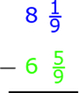# The quantity

Find the quantity of six times 3 1/2 over 2.

x =  10 12 = 21/2

### Step-by-step explanation:Did you find an error or inaccuracy? Feel free to write us. Thank you!

Showing 1 comment:
Math Student
The quantity of six times 3 1/2 over 2

Convert 3 ½ into improper fraction.

3 ½ → 7/2

Convert into Multiplication Process

Lowest Term

= 10 ½

Tips for related online calculators
Need help calculating sum, simplifying, or multiplying fractions? Try our fraction calculator.
Need help with mixed numbers? Try our mixed-number calculator.